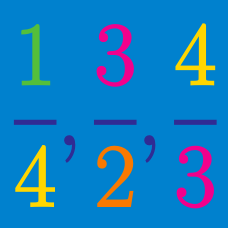Number Theory

# Rational Numbers: Level 2 Challenges

What is $0.1212 \ldots$ in fractional form?

If $\frac{1}{21}$ equals the repeating decimal $0.0476190476190...$, what is the $51^{st}$digit after the decimal point of the repeating decimal?

$\frac{n}{ 810} = 0.\overline{d25} = 0.d25d25d25d25\ldots$

For which positive integer $n$, will $d$ be a digit?

$\large \displaystyle {0. \overline{42}-0.\overline{35}= \, ?}$


Note: $0.\overline{ab}=0.abababab \ldots$

Is $0.285714285714\overline {285714}$ rational?


Note: The notation $“\, \overline{285714}"$ indicates that these digits in the decimal are being repeated. For example, $0.1\overline{2} = 0.12222 \ldots.$

×